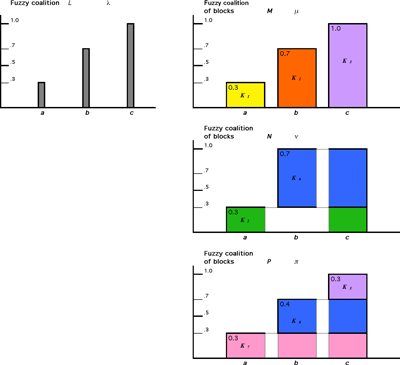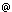# Models of Multilateral Cooperative Behaviour

by Milan Mares

The concept of artificial intelligence includes the possibility of cooperation between its representatives (eg robots, complex man-machine systems or other structures), where each is attempting to maximize the values of its goal function. Such an endeavour is formally defined as a cooperative game in which players coordinate their strategies in order to maximize the individual pay-offs for each of them. After a long period of investigation into deterministic games, our attention has recently been focused on games in which the cooperation is distributed among several parallel interests.

The classical theory of cooperative games assumes that, after the negotiation period, the set of players separates into disjoint coalitions and each player participates in exactly one of them. This assumption is not realistic - in real communities with non-trivial structure, regardless of whether their members are human beings or coordinated artificial decision-makers, each member simultaneously follows several goals, distributing its 'power' among them. To achieve these goals, the player cooperates with several groups of partners. Such distributed cooperation is usually modelled by fuzzy coalitions, which have been investigated since the early eighties.

The classical model defines fuzzy coalition as a fuzzy subset of the set of all players. This approach displays many advantages, but also has some problems. The fundamental nature of these becomes evident when the formal model is to be compared with its intuitive interpretation. For example, such a concept of fuzzy coalitions practically excludes their disjointness (the classical fuzzy set theoretical disjointness practically eliminates the multilaterality of cooperation). Consequently, it eliminates also the principle of superadditivity and related game-theoretical concepts specifying the distribution of the coalitional pay-offs.

This recent research was supported by grants from the Grant Agency of the Czech Republic No. 402/04/1026 and the Grant Agency of the Academy of Sciences of CR No. A1075301. Its goal was to suggest an alternative model of cooperative behaviour with distributed cooperation, better reflecting the superadditivity and related properties of fuzzy coalitions. The main motivation was to find an adequate model of the complex structure of the cooperative relations divided into several fields of interests.To illustrate the difference between the subtlety of both concepts of fuzzy coalitions, let us consider a simple three-player game with players a, b, c and possible deterministic coalitions (blocks) K0 = φ, K1 = {a}, K2 = {b}, K3 = {c}, K4 = {a, b}, K5 = {a, c}, K6 = {b, c}, K7 = {a, b, c}. Consider a fuzzy coalition L with membership function λ, where λ(a) = 0.3 , λ(b) = 0.7, λ(c) = 1.0. Then there exist several fuzzy coalitions of the new (block-wise) type representing the above activities of individual players. For example, M with membership function m , where μ(K!) = μ(a) = 0.3, μ(K2) = l(b) = 0.7, μ(K3) = λ(c) = 1.0; N with memberships ν , where ν(K5) = 0.3, ν(K6) = 0.7; or P with π, where π(K7) = 0.3, π(K6) = 04, π(K3) and some others. Each represents a different structure of the concern of players participating in coalition L via particular blocks. The total concern of each player is unchanged even if its distribution among blocks varies.

The suggested alternative approach is based on the definition of a fuzzy coalition as a fuzzy subclass of the class of all deterministic coalitions (ie of all subsets of the set of all players; in this model, called 'blocks'). This approach reflects the fact that players usually divide their 'power' (investments, energy, social contacts, authority etc) among several interests in which they cooperate with equivalent (similarly motivated) partners. These blocks enter the conflict as relatively compact units and the total incomes of particular players comprise the sum of their incomes from each block. Each block can be active in several fuzzy coalitions. In this sense, the disjointness concept can be simplified: the simultaneous participation of a player in several fuzzy coalitions (intermediated via his participation in different blocks) does not impair their disjointness if he acts in them as a member of different blocks.

This model displays certain advantages. One of them is the formal simplicity of the definition of superadditivity, subadditivity and convexity, with interesting consequences for the concept and properties of the core and other solutions. Another, perhaps even more interesting advantage is that the alternative model offers much more sophisticated tools for the characterization of the cooperation. It is easy to see that the classical fuzzy coalitions can be transformed into several fuzzy coalitions of the new type. They reflect in some sense similar relations among players, but offer many more details regarding the subtle differences of their inner structure.

E-mail: maresutia.cas.cz